# Frank Solutions Class 9 Maths Chapter 23 Graphical Representation of Statistical Data

Frank Solutions for Class 9 Maths Chapter 23 Graphical Representation of Statistical Data provide students with 100% accurate answers in a lucid manner. Students who have doubts related to the concepts and topics covered in this chapter are advised to solve Frank Solutions so that they can clear their doubts quickly. Practising Frank Solutions on a regular basis will enhance their problem-solving skills, which are essential to boost exam preparation.

Chapter 23, Graphical Representation of Statistical Data, has problems which give information on data using graphs. The way of analysing numerical data is known as a graphical representation. Referring to the Frank Solutions, while solving the textbook questions, will help students to clear their doubts instantly as well as score good marks in the annual exams. Students can access Frank Solutions for Class 9 Maths Chapter 23 Graphical Representation of Statistical Data PDF from the link provided below.

## Frank Solutions for Class 9 Maths Chapter 23 Graphical Representation of Statistical Data Download PDF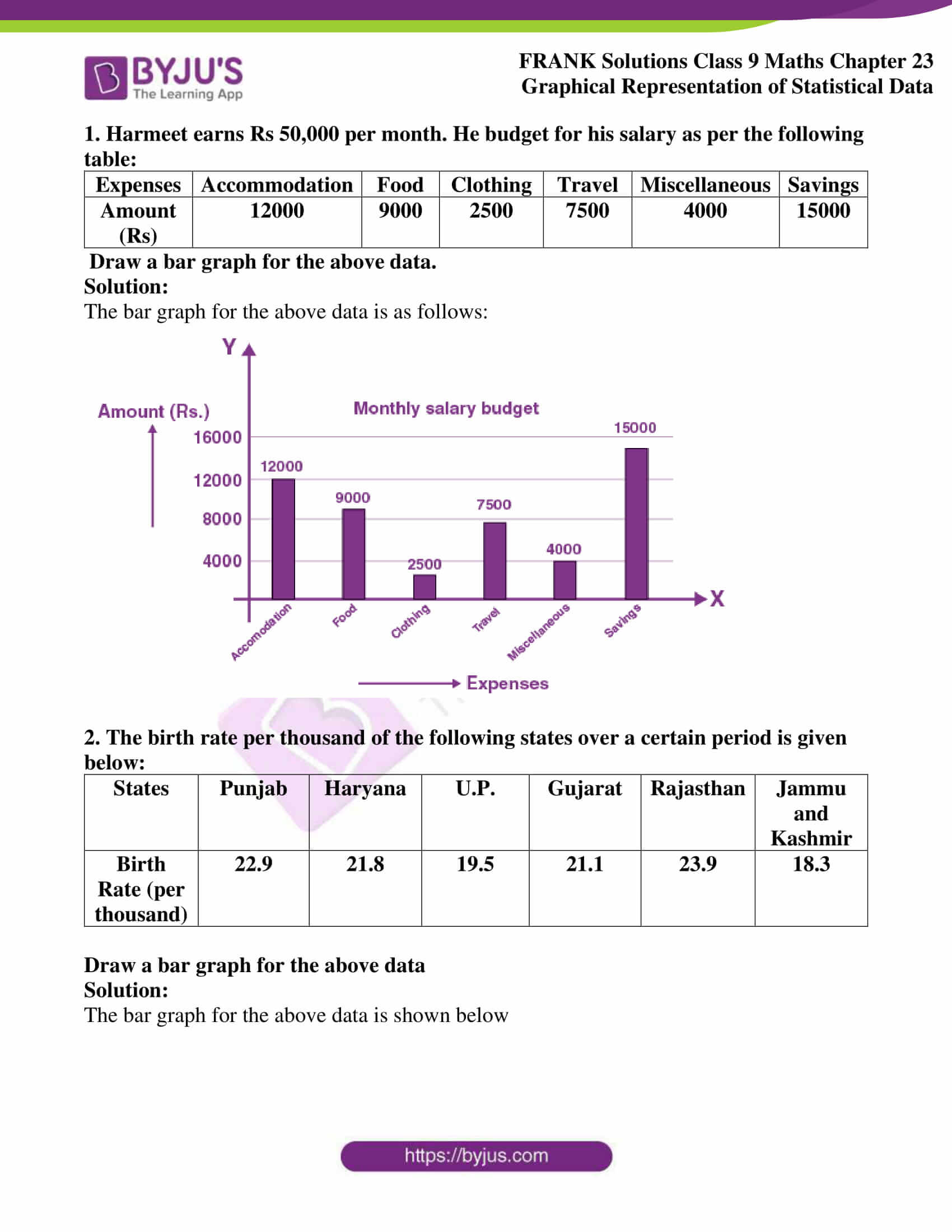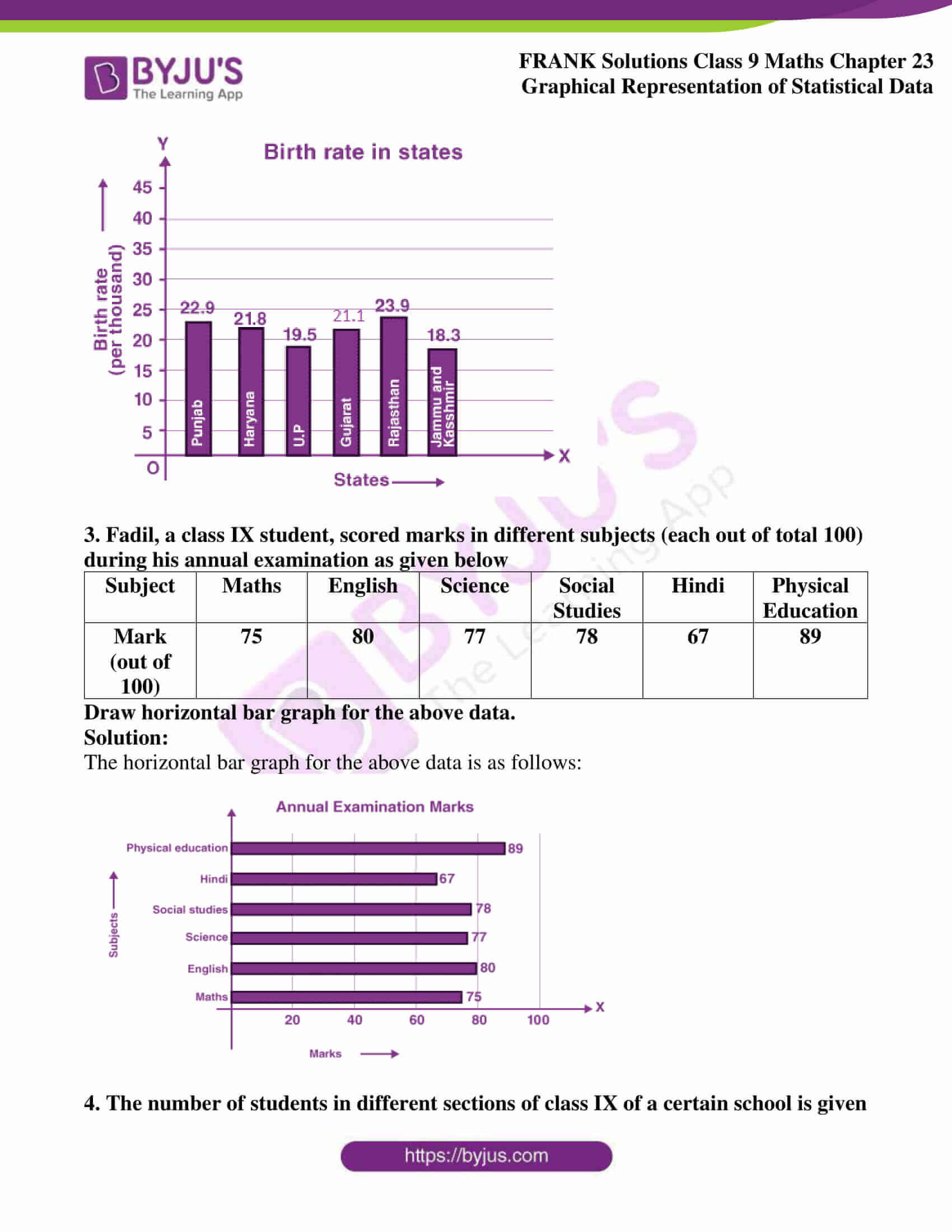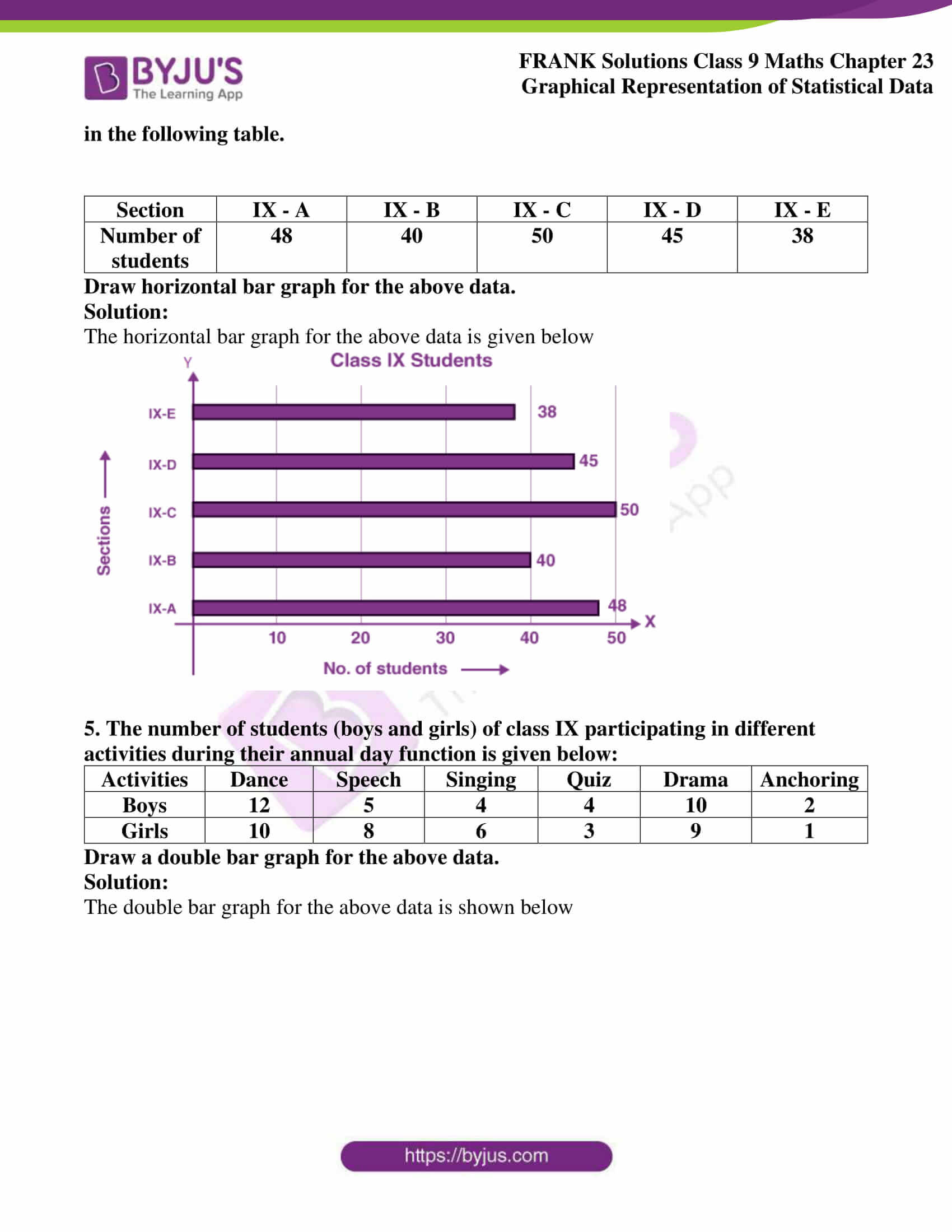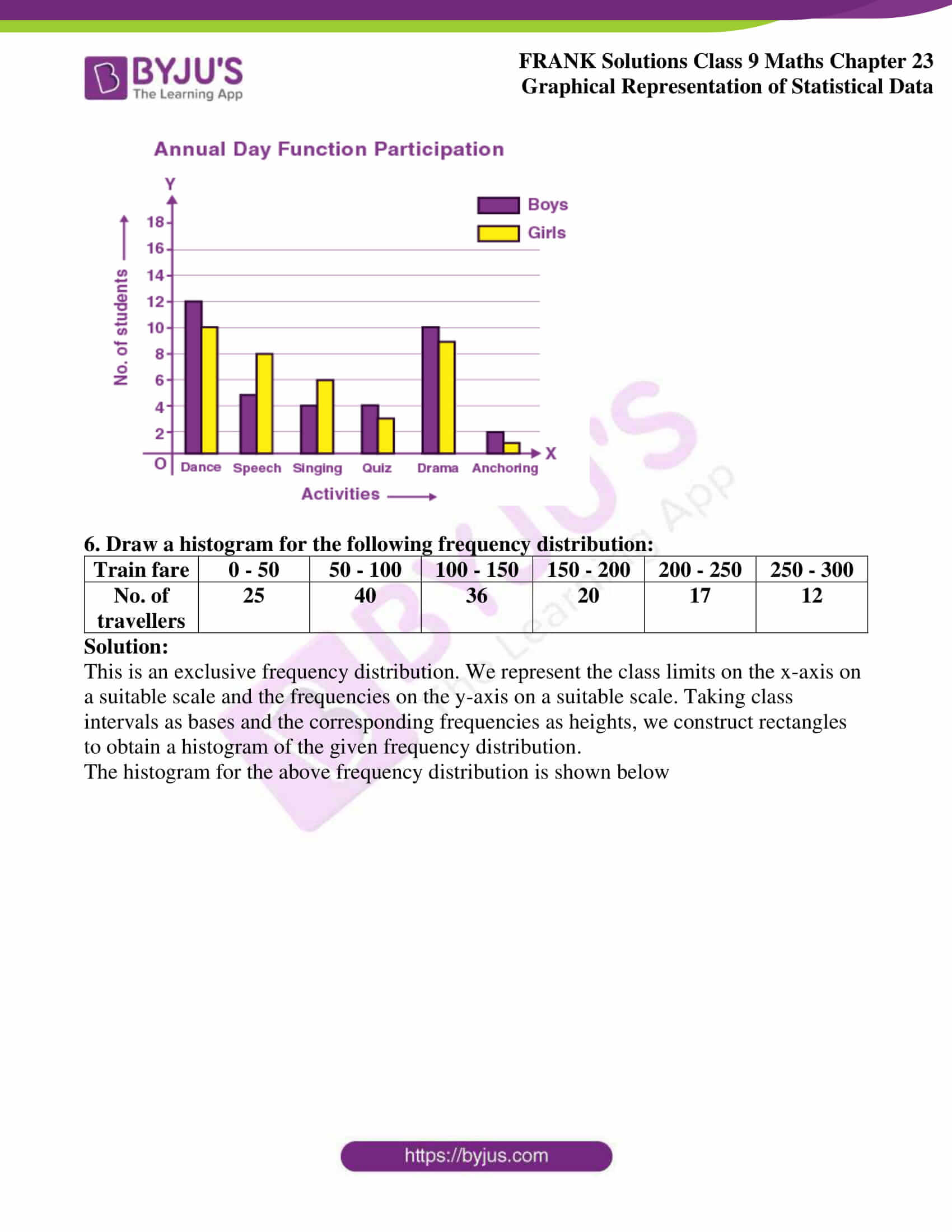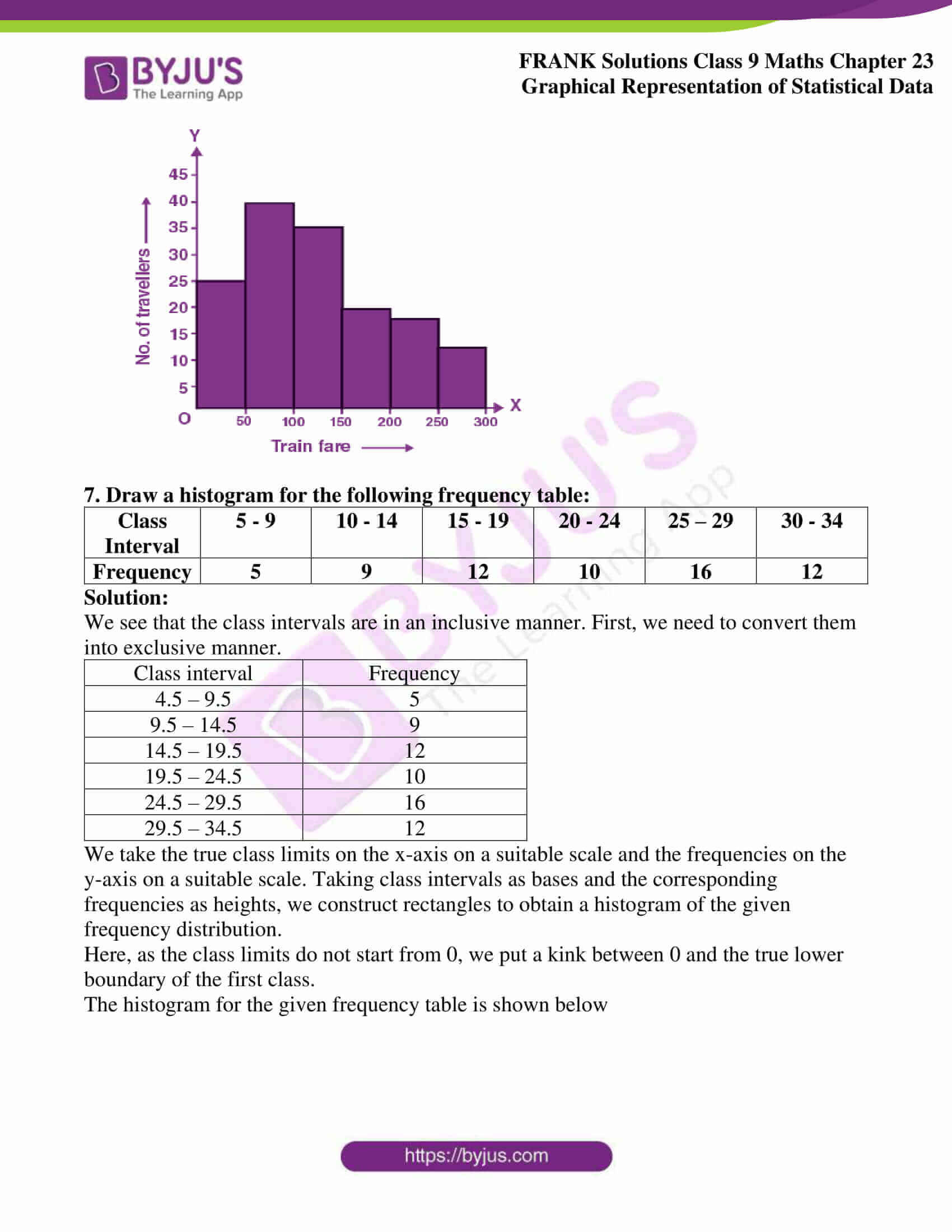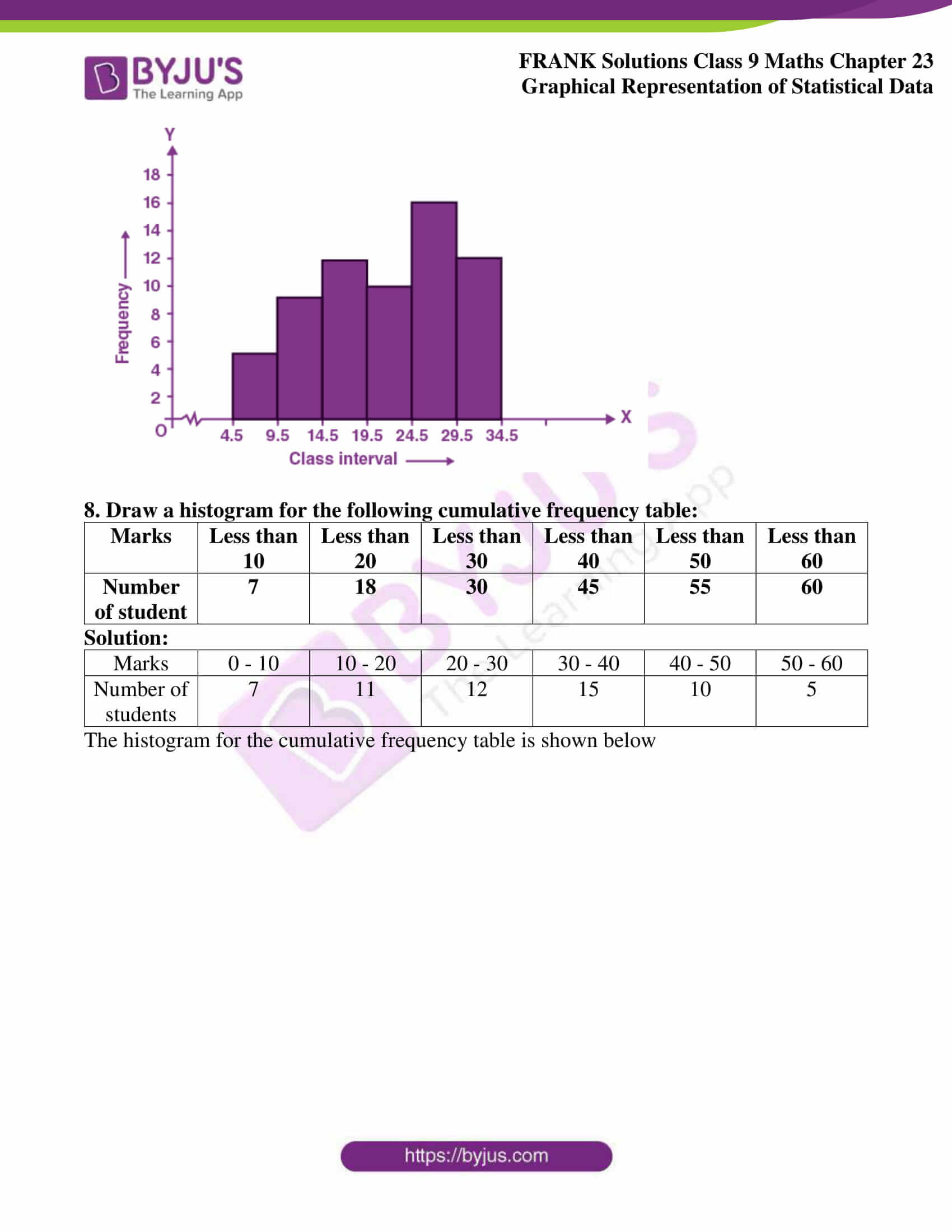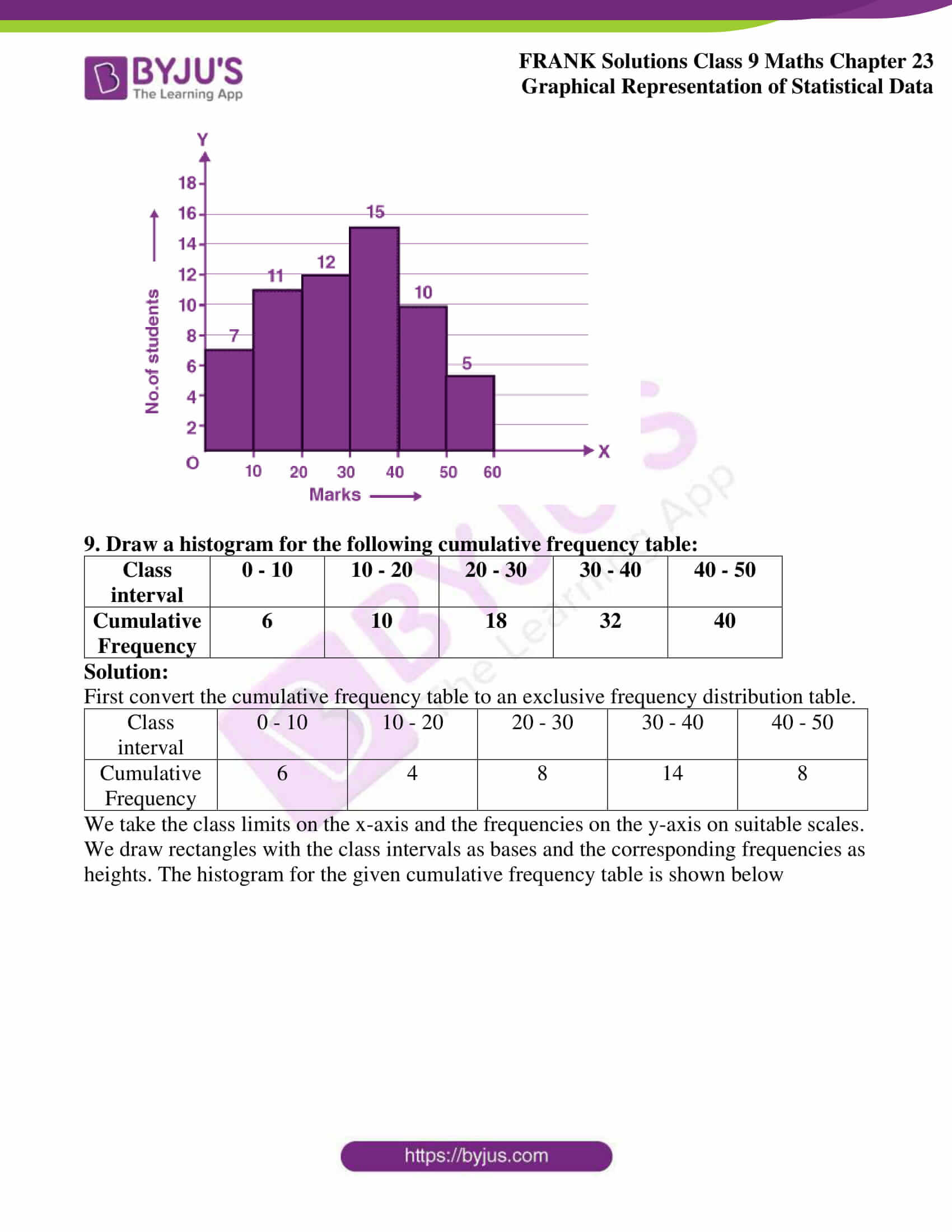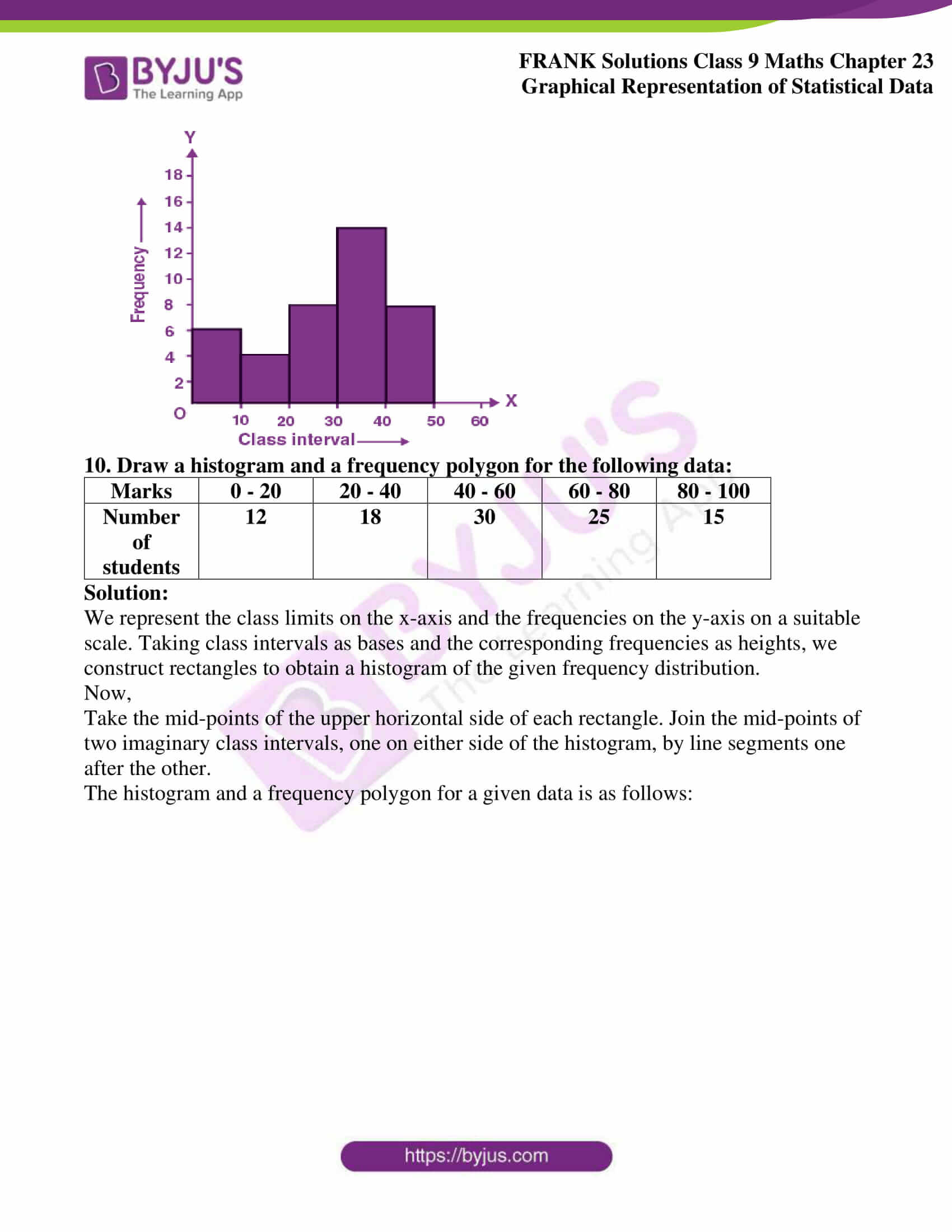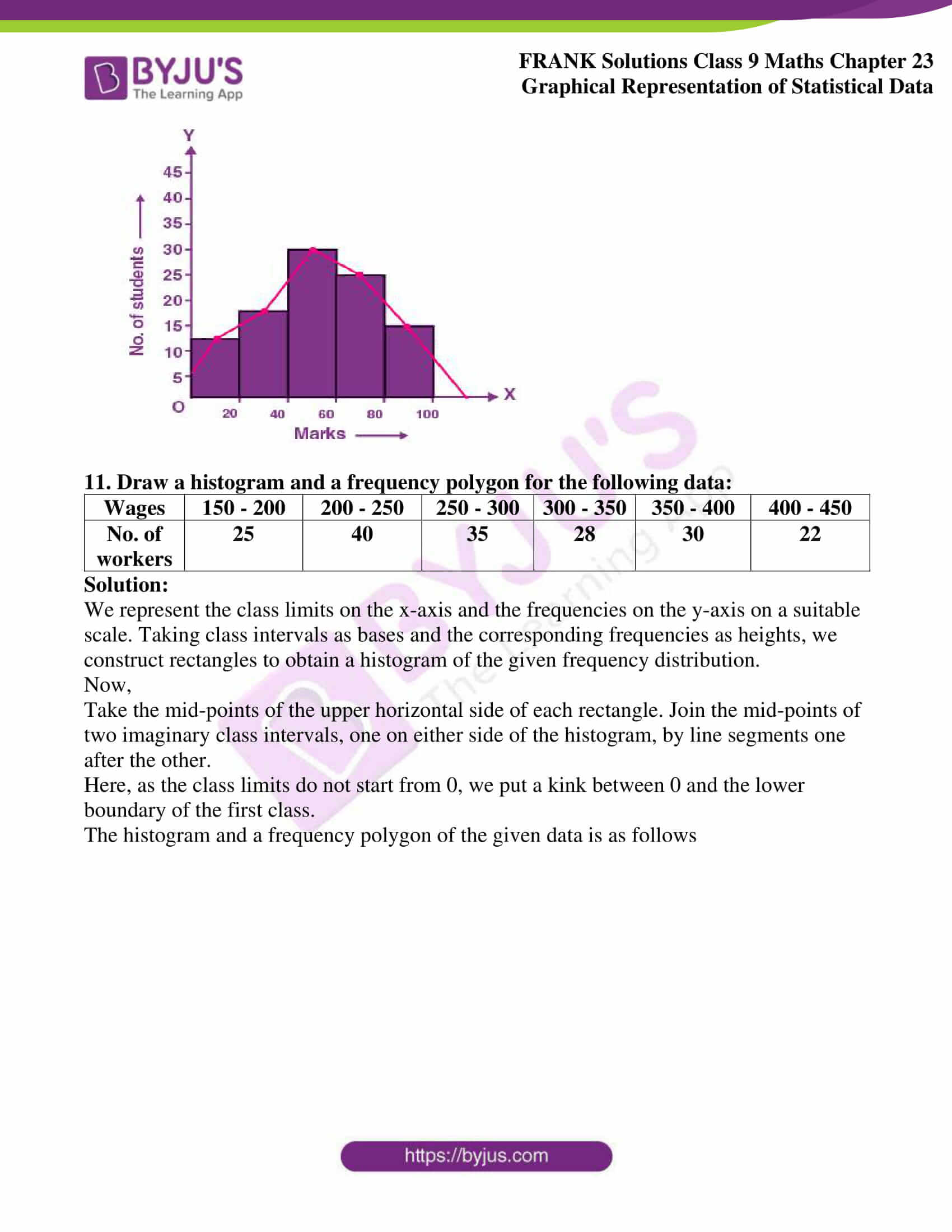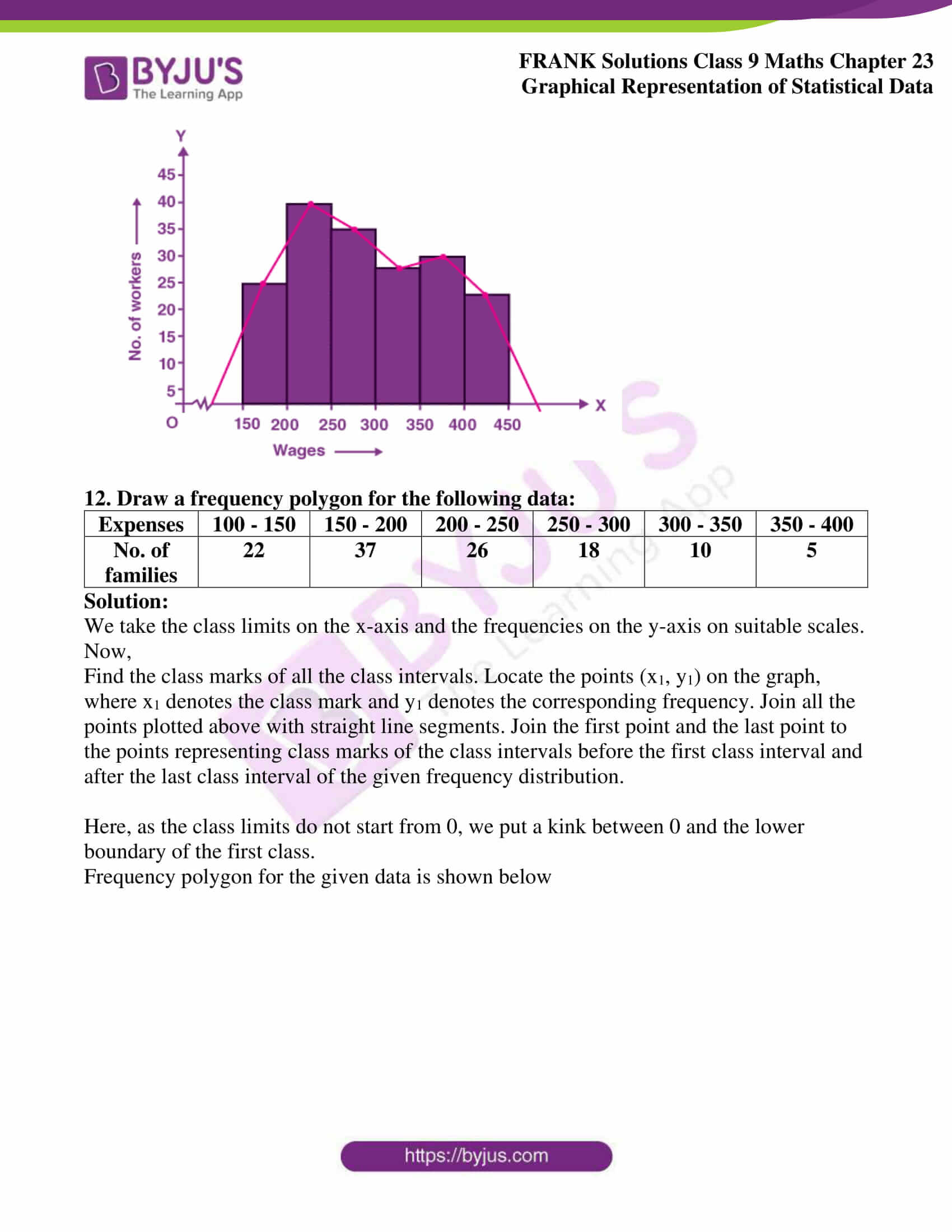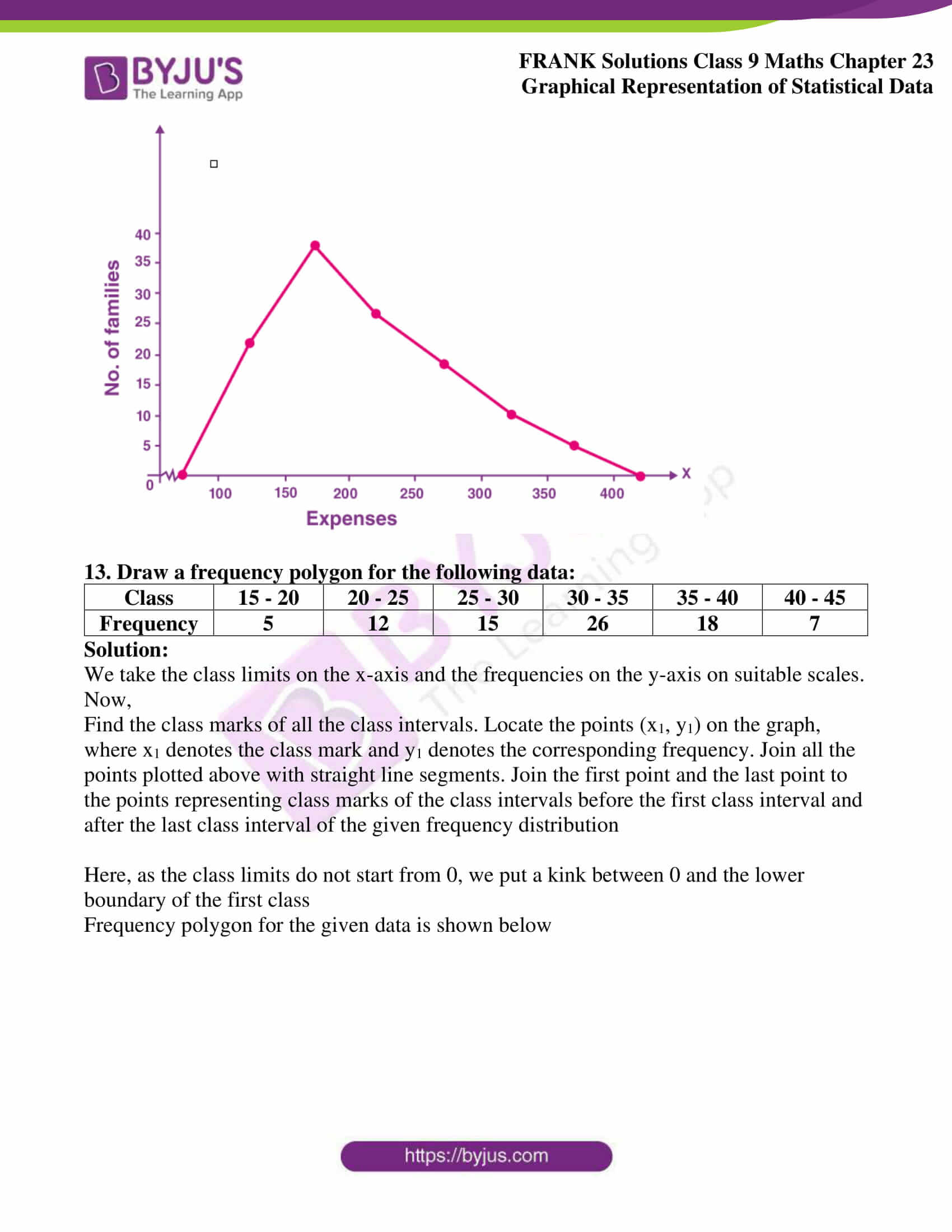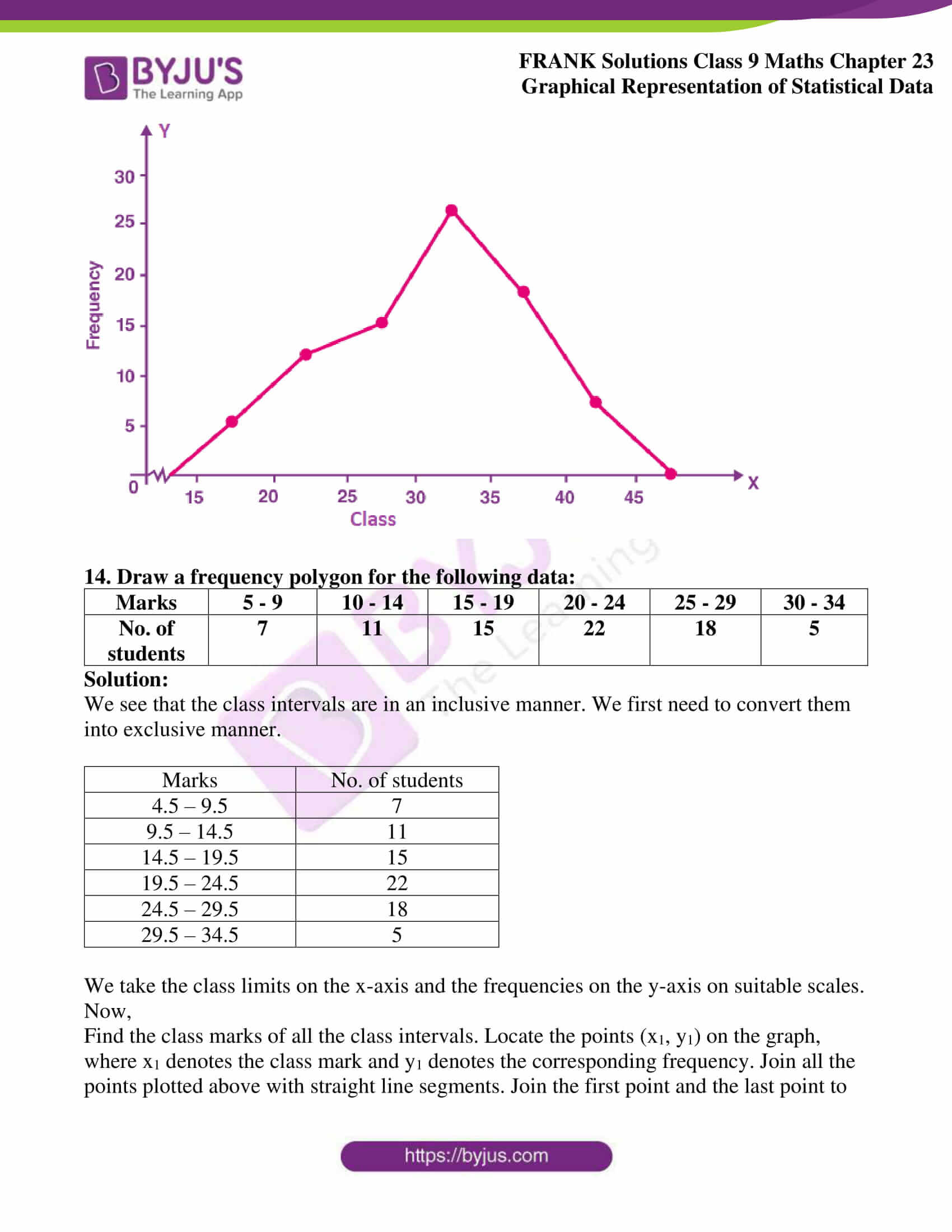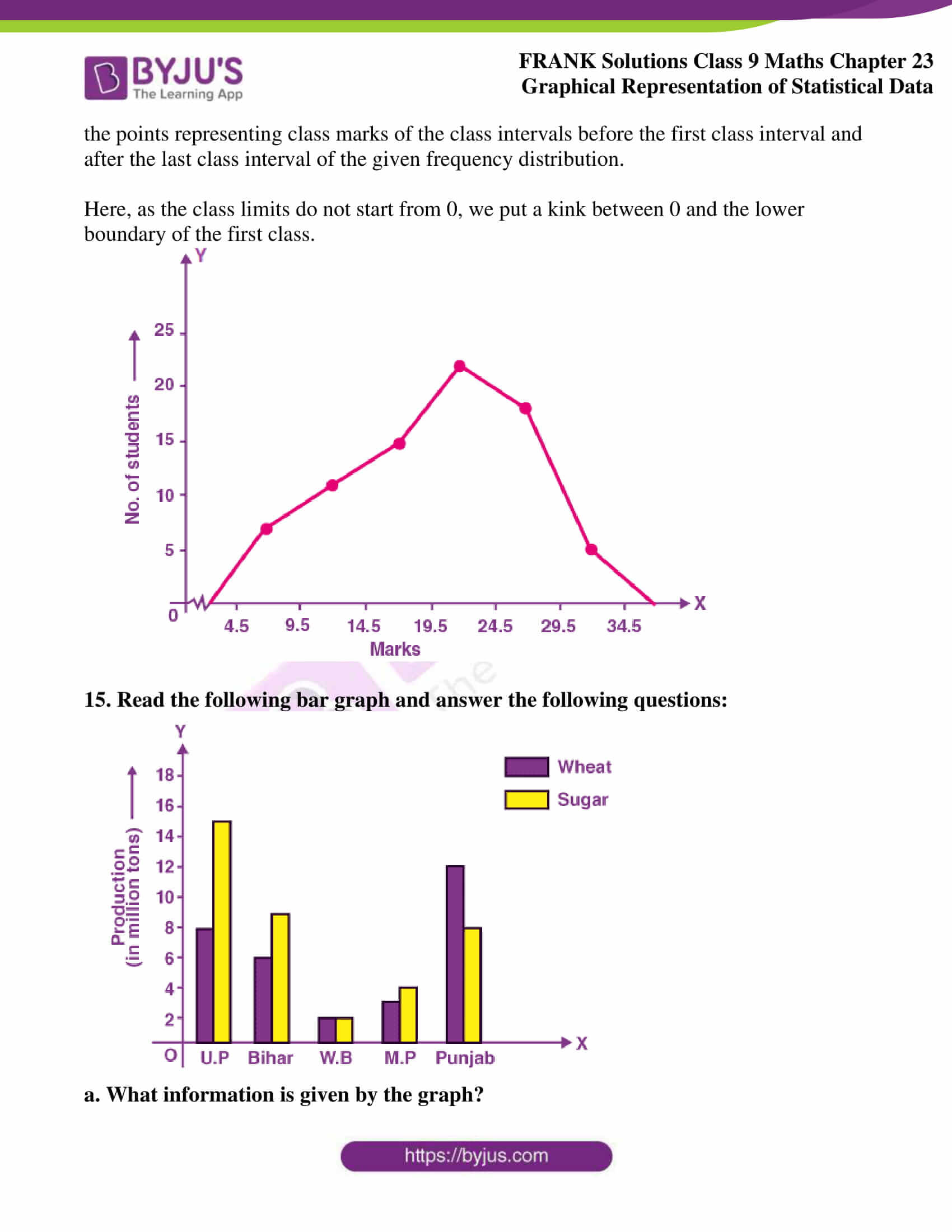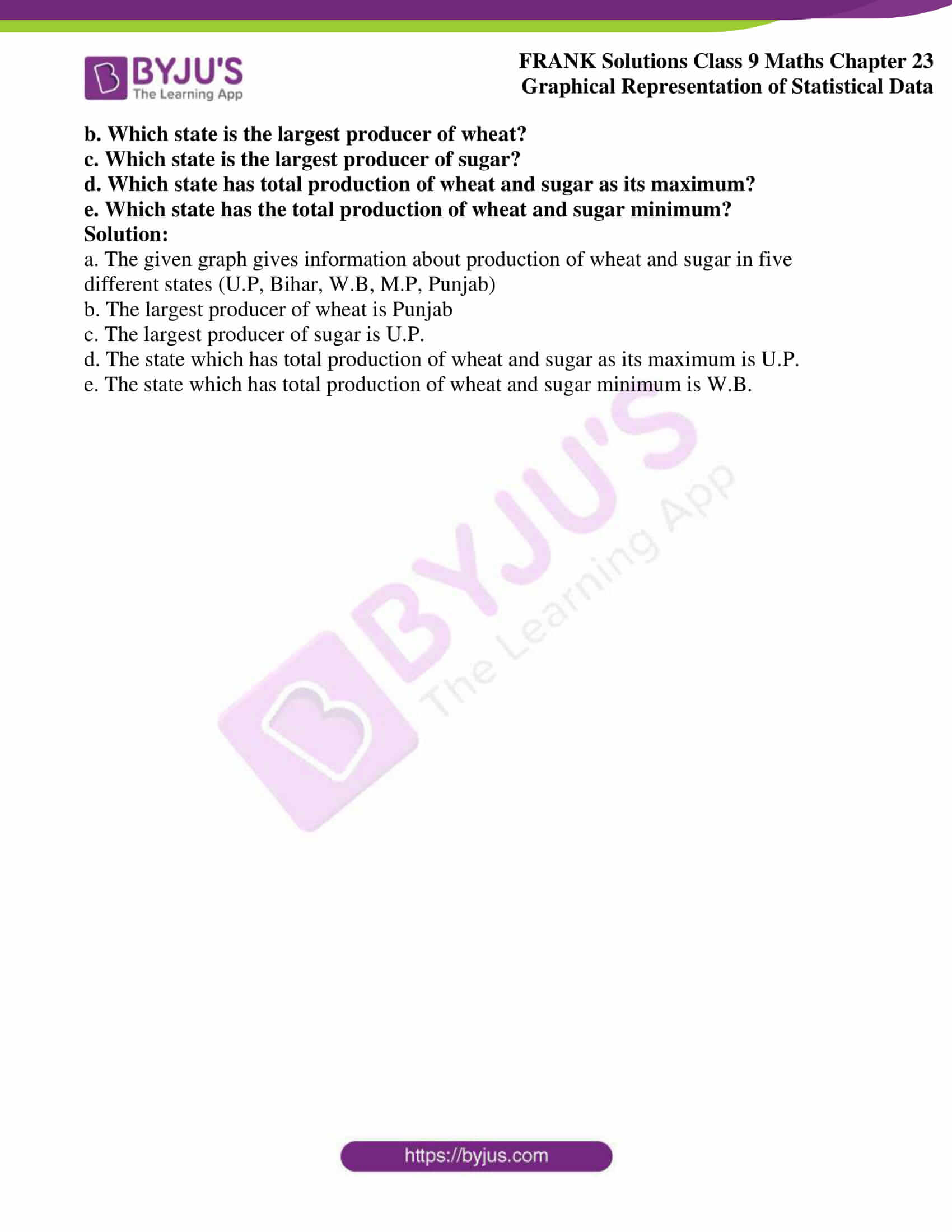## Access Frank Solutions for Class 9 Maths Chapter 23 Graphical Representation of Statistical Data

1. Harmeet earns Rs 50,000 per month. He budgeted for his salary as per the following table:

 Expenses Accommodation Food Clothing Travel Miscellaneous Savings Amount (Rs) 12000 9000 2500 7500 4000 15000

Draw a bar graph for the above data.

Solution:

The bar graph for the above data is as follows: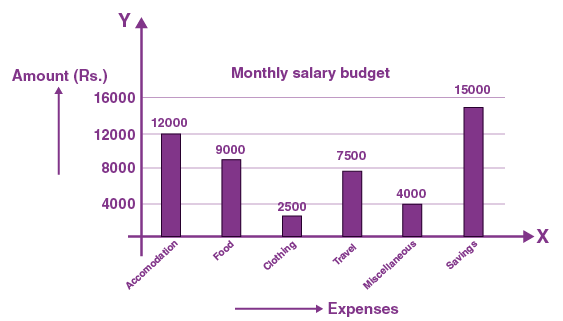2. The birth rate per thousand of the following states over a certain period is given below:

 States Punjab Haryana U.P. Gujarat Rajasthan Jammu and Kashmir Birth Rate (per thousand) 22.9 21.8 19.5 21.1 23.9 18.3

Draw a bar graph for the above data

Solution:

The bar graph for the above data is shown below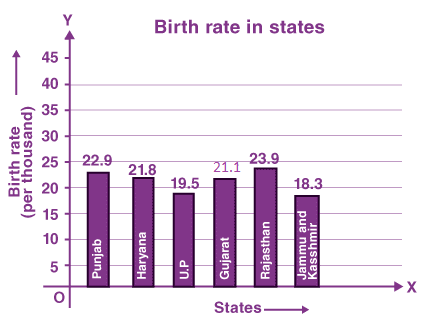3. Fadil, a class IX student, scored marks in different subjects (each out of total 100) during his annual examination, as given below

 Subject Maths English Science Social Studies Hindi Physical Education Mark (out of 100) 75 80 77 78 67 89

Draw a horizontal bar graph for the above data.

Solution:

The horizontal bar graph for the above data is as follows: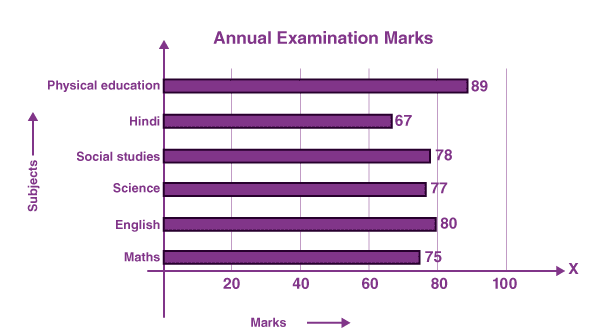4. The number of students in different sections of class IX of a certain school is given in the following table.

 Section IX – A IX – B IX – C IX – D IX – E Number of students 48 40 50 45 38

Draw a horizontal bar graph for the above data.

Solution:

The horizontal bar graph for the above data is given below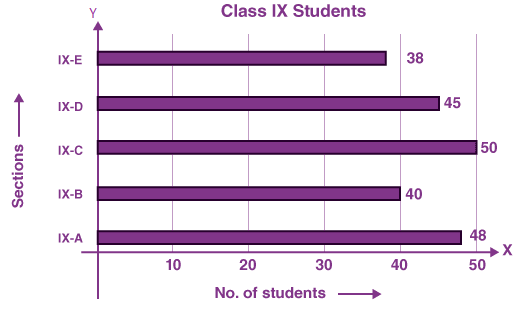5. The number of students (boys and girls) of class IX participating in different activities during their annual day function is given below:

 Activities Dance Speech Singing Quiz Drama Anchoring Boys 12 5 4 4 10 2 Girls 10 8 6 3 9 1

Draw a double bar graph for the above data.

Solution:

The double bar graph for the above data is shown below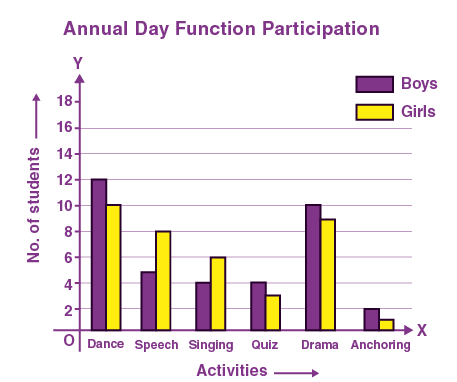6. Draw a histogram for the following frequency distribution:

 Train fare 0 – 50 50 – 100 100 – 150 150 – 200 200 – 250 250 – 300 No. of travellers 25 40 36 20 17 12

Solution:

This is an exclusive frequency distribution. We represent the class limits on the x-axis on a suitable scale and the frequencies on the y-axis on a suitable scale. Taking class intervals as bases and the corresponding frequencies as heights, we construct rectangles to obtain a histogram of the given frequency distribution.

The histogram for the above frequency distribution is shown below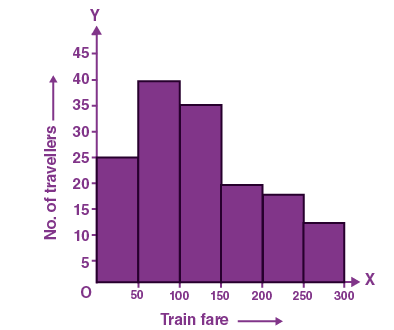7. Draw a histogram for the following frequency table:

 Class Interval 5 – 9 10 – 14 15 – 19 20 – 24 25 – 29 30 – 34 Frequency 5 9 12 10 16 12

Solution:

We see that the class intervals are in inclusive manner. First, we need to convert them to an exclusive manner.

 Class interval Frequency 4.5 – 9.5 5 9.5 – 14.5 9 14.5 – 19.5 12 19.5 – 24.5 10 24.5 – 29.5 16 29.5 – 34.5 12

We take the true class limits on the x-axis on a suitable scale and the frequencies on the y-axis on a suitable scale. Taking class intervals as bases and the corresponding frequencies as heights, we construct rectangles to obtain a histogram of the given frequency distribution.

Here, as the class limits do not start from 0, we put a kink between 0 and the true lower boundary of the first class.

The histogram for the given frequency table is shown below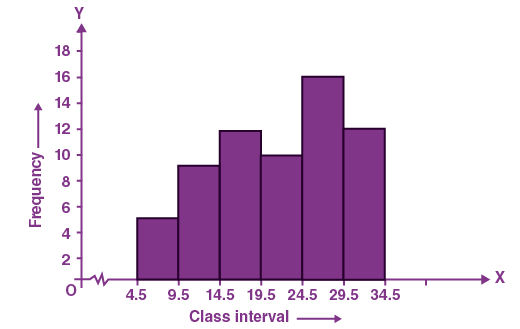8. Draw a histogram for the following cumulative frequency table:

 Marks Less than 10 Less than 20 Less than 30 Less than 40 Less than 50 Less than 60 Number of students 7 18 30 45 55 60

Solution:

 Marks 0 – 10 10 – 20 20 – 30 30 – 40 40 – 50 50 – 60 Number of students 7 11 12 15 10 5

The histogram for the cumulative frequency table is shown below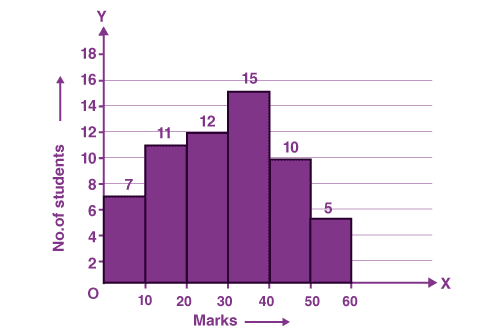9. Draw a histogram for the following cumulative frequency table:

 Class interval 0 – 10 10 – 20 20 – 30 30 – 40 40 – 50 Cumulative Frequency 6 10 18 32 40

Solution:

First, convert the cumulative frequency table to an exclusive frequency distribution table.

 Class interval 0 – 10 10 – 20 20 – 30 30 – 40 40 – 50 Cumulative Frequency 6 4 8 14 8

We take the class limits on the x-axis and the frequencies on the y-axis on suitable scales. We draw rectangles with the class intervals as bases and the corresponding frequencies as heights. The histogram for the given cumulative frequency table is shown below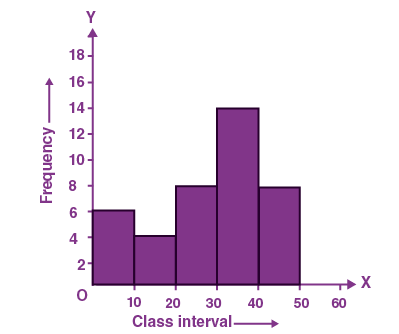10. Draw a histogram and a frequency polygon for the following data:

 Marks 0 – 20 20 – 40 40 – 60 60 – 80 80 – 100 Number of students 12 18 30 25 15

Solution:

We represent the class limits on the x-axis and the frequencies on the y-axis on a suitable scale. Taking class intervals as bases and the corresponding frequencies as heights, we construct rectangles to obtain a histogram of the given frequency distribution.

Now,

Take the mid-point of the upper horizontal side of each rectangle. Join the mid-points of two imaginary class intervals, one on either side of the histogram, by line segments one after the other.

The histogram and a frequency polygon for a given data are as follows: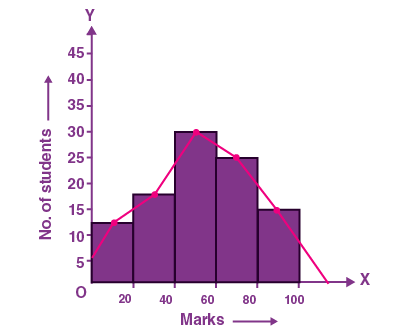11. Draw a histogram and a frequency polygon for the following data:

 Wages 150 – 200 200 – 250 250 – 300 300 – 350 350 – 400 400 – 450 No. of workers 25 40 35 28 30 22

Solution:

We represent the class limits on the x-axis and the frequencies on the y-axis on a suitable scale. Taking class intervals as bases and the corresponding frequencies as heights, we construct rectangles to obtain a histogram of the given frequency distribution.

Now,

Take the mid-point of the upper horizontal side of each rectangle. Join the mid-points of two imaginary class intervals, one on either side of the histogram, by line segments one after the other.

Here, as the class limits do not start from 0, we put a kink between 0 and the lower boundary of the first class.

The histogram and a frequency polygon of the given data are as follows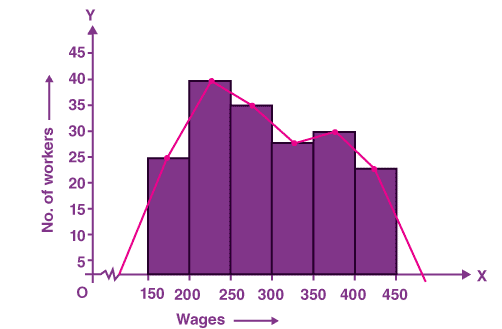12. Draw a frequency polygon for the following data:

 Expenses 100 – 150 150 – 200 200 – 250 250 – 300 300 – 350 350 – 400 No. of families 22 37 26 18 10 5

Solution:

We take the class limits on the x-axis and the frequencies on the y-axis on suitable scales.

Now,

Find the class marks of all the class intervals. Locate the points (x1, y1) on the graph, where x1 denotes the class mark and y1 denotes the corresponding frequency. Join all the points plotted above with straight-line segments. Join the first point and the last point to the points representing class marks of the class intervals before the first class interval and after the last class interval of the given frequency distribution.

Here, as the class limits do not start from 0, we put a kink between 0 and the lower boundary of the first class.

The frequency polygon for the given data is shown below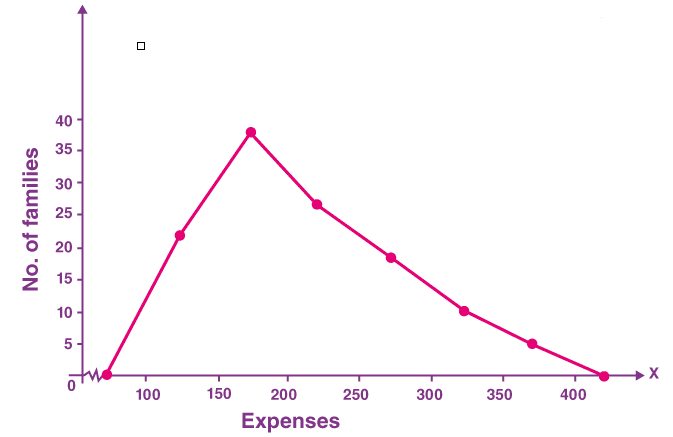13. Draw a frequency polygon for the following data:

 Class 15 – 20 20 – 25 25 – 30 30 – 35 35 – 40 40 – 45 Frequency 5 12 15 26 18 7

Solution:

We take the class limits on the x-axis and the frequencies on the y-axis on suitable scales.

Now,

Find the class marks of all the class intervals. Locate the points (x1, y1) on the graph, where x1 denotes the class mark and y1 denotes the corresponding frequency. Join all the points plotted above with straight-line segments. Join the first point and the last point to the points representing class marks of the class intervals before the first class interval and after the last class interval of the given frequency distribution

Here, as the class limits do not start from 0, we put a kink between 0 and the lower boundary of the first class

The frequency polygon for the given data is shown below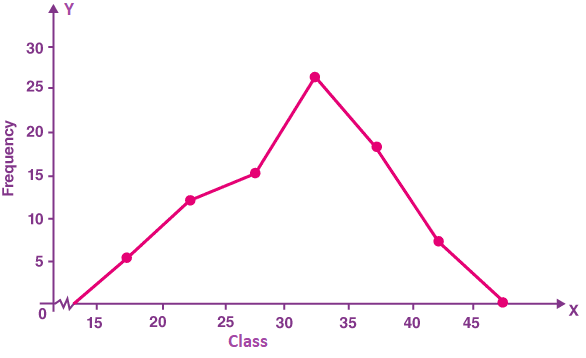14. Draw a frequency polygon for the following data:

 Marks 5 – 9 10 – 14 15 – 19 20 – 24 25 – 29 30 – 34 No. of students 7 11 15 22 18 5

Solution:

We see that the class intervals are in inclusive manner. First, we need to convert them to an exclusive manner.

 Marks No. of students 4.5 – 9.5 7 9.5 – 14.5 11 14.5 – 19.5 15 19.5 – 24.5 22 24.5 – 29.5 18 29.5 – 34.5 5

We take the class limits on the x-axis and the frequencies on the y-axis on suitable scales.

Now,

Find the class marks of all the class intervals. Locate the points (x1, y1) on the graph, where x1 denotes the class mark and y1 denotes the corresponding frequency. Join all the points plotted above with straight-line segments. Join the first point and the last point to the points representing class marks of the class intervals before the first class interval and after the last class interval of the given frequency distribution.

Here, as the class limits do not start from 0, we put a kink between 0 and the lower boundary of the first class.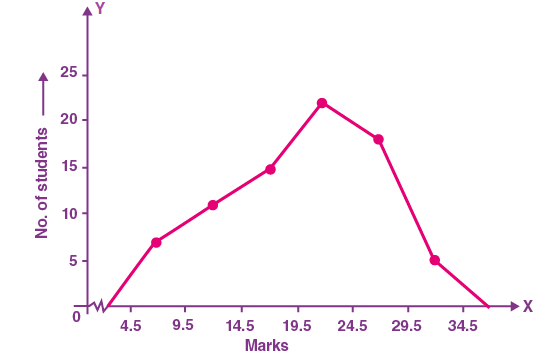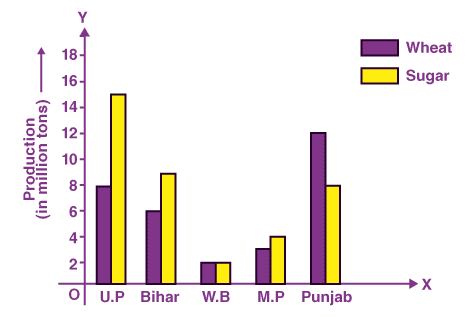a. What information is given by the graph?

b. Which state is the largest producer of wheat?

c. Which state is the largest producer of sugar?

d. Which state has the total production of wheat and sugar as its maximum?

e. Which state has the total production of wheat and sugar minimum?

Solution:

a. The given graph gives information about the production of wheat and sugar in five different states (U.P, Bihar, W.B, M.P, Punjab)

b. The largest producer of wheat is Punjab

c. The largest producer of sugar is U.P.

d. The state which has the total production of wheat and sugar as its maximum is U.P.

e. The state which has the total production of wheat and sugar minimum is W.B.